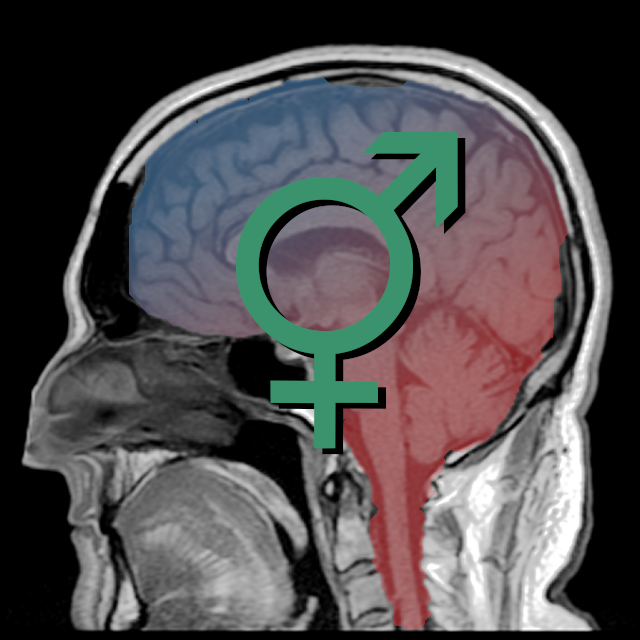# BIOLOGICAL SEX CLASSIFICATION USING MRI

This trained classifier is intended to predict the biological sex on the basis of an MRI image. It has a measured balanced accuracy of 94.01% on a validation set of N=351.

## Training

Individualized prediction of the biological sex was assessed by the use of a support vector classifier as implemented in the Scikit-learn toolbox. CAT12 whole-brain gray matter images were used as a classifier input.

To reduce dimensionality by preserving maximal localized morphometric differences, gray matter images were resliced to a voxel size of 3 mm × 3 mm × 3 mm. To strictly separate the training process from the evaluation a random validation set of 20 % (N=351, female=219, male=132) was selected, which was not used during classifier training and testing. The remaining data set of N=1402 subjects was balanced with a random undersampling procedure (N=1218, female=609, male=609) and used in a 10-fold split procedure resulting in balanced training sets of 1096 subjects in each stepfold.

Then we conducted a principal component analysis to further reduce the dimensionality of the data. The maximum number of principal components is limited to 1096, the number of subjects resulting from the 10-fold split. We carried out a Bayes statistic based hyperparameter optimization for the SVC (Scikit-Optimize) nested in the 10-fold cross-validation.

The parameter search included choice of the kernel (radial basis function (rbf) or linear), the C parameter (10^(-2) to 10^2, non-discrete log-scale), which influences penalties for misclassification, and the gamma parameter (10^(-6) to 10, non-discrete log-sale), influencing the curvature of the decision boundary.

In this iterative Bayes approach a total of 100 parameter combinations were evaluated. Quality and classifier performance are reported by area under the ROC curve (AUC).

## Results

The training of the classifier lead to two results. The first result was the estimation of a hyperparameter set, determined with the Bayes optimization method. The hyperparameter optimization estimated a rbf kernel, C=27.3 and gamma=2.4 × 10-05 for the SVM as optimal approximation for the present problem.

The second result was the classification outcome of the held out 20% validation set, which gave a performance indication for the trained classifier. The balanced accuracy for the validation set classification was 94.01%.

### Usage

See file use_pretrained_classifier.py code file contained in archive